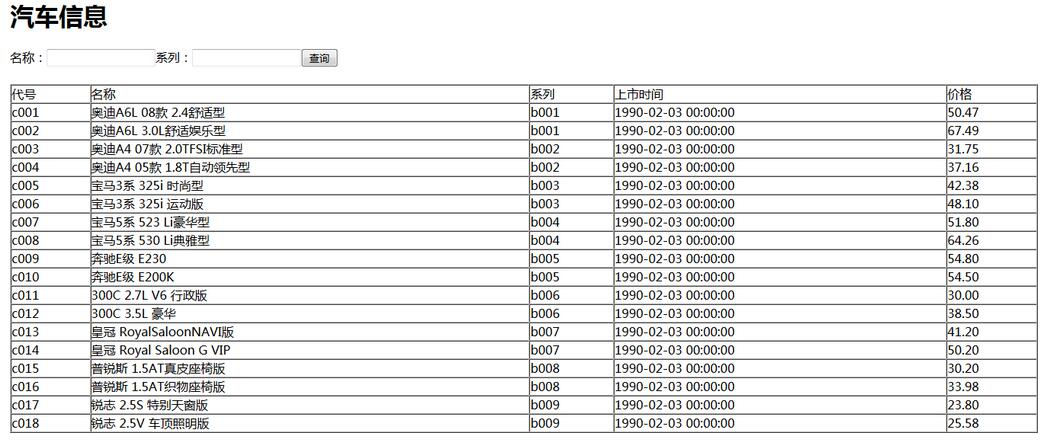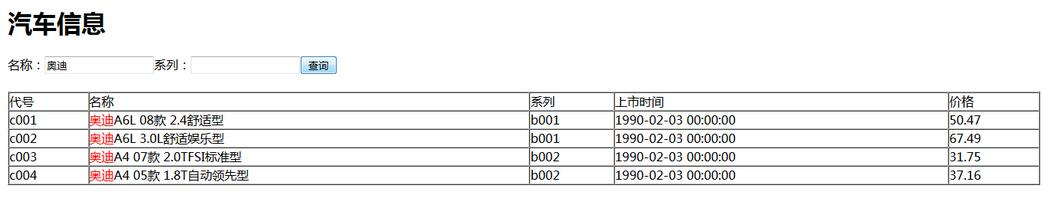# php实现查询功能（数据访问）

更新时间：2017年05月23日 15:04:33   作者：ChrissZhao我要评论```<body>
<h1>汽车信息</h1>
<?php
require"DBDA.class1.php";//\$type 代表SQL语句的类型，0代表增删改，1代表查询
\$db = new DBDA();

//如果没有提交数据，显示所有
//如果有提交数据，根据关键字查询显示
\$name = "";
\$tj1 = " 1=1 ";//第一个条件，对应名称，注意空格
\$tj2 = " 1=1 ";//第二个条件，对应系列，注意空格
if(!empty(\$_POST["name"]))
{
\$name = \$_POST["name"];
\$tj1 = " name like '%{\$name}%'";
}
if(!empty(\$_POST["brand"]))
{
\$brand = \$_POST["brand"];
\$tj2 = " brand like '%{\$brand}%'";
}
//总条件
\$tj ="{\$tj1} and {\$tj2}";
\$sql = "select * from car where ".\$tj;//注意where后空格
/*echo \$sql;*/
?>
<form action="chaxun.php" method="post">
<div>名称：<input type="text" name="name" value="<?php echo \$name ?>"/>系列：<input type="text" name="brand"/><input type="submit" value="查询" /></div>
</form>
<br/>
<table width="100%" border="1" cellpadding="0" cellspacing="0">
<tr>
<td>代号</td>
<td>名称</td>
<td>系列</td>
<td>上市时间</td>
<td>价格</td>
</tr>
<?php

//只适用于单条件查询
/*\$sql = "select * from car";
\$name="";
if(!empty(\$_POST["name"]))
{
\$name = \$_POST["name"];
\$sql = "select * from car where name like '%{\$name}%' ";
}*/

\$arr = \$db->query(\$sql);
foreach(\$arr as \$v)
{
\$str = str_replace(\$name,"<span style='color:red'>{\$name}</span>",\$v);
echo "<tr>
<td>{\$v}</td>
<td>{\$str}</td>
<td>{\$v}</td>
<td>{\$v}</td>
<td>{\$v}</td>
</tr>";
}
?>
</table>
</body>
</html>
```

```<?php
class DBDA
{
public \$host = "localhost";
public \$uid = "root";
public \$pwd = "123";
public \$dbname = "newssystem";
//执行SQL语句返回相应的结果
//\$sql 要执行的SQL语句
//\$type 代表SQL语句的类型，0代表增删改，1代表查询
function query(\$sql,\$type=1)
{
\$db = new MySQLi(\$this->host,\$this->uid,\$this->pwd,\$this->dbname);

\$result = \$db->query(\$sql);

if(\$type)
{
//如果是查询，显示数据
return \$result->fetch_all();
}
else
{
//如果是增删改，返回true或者false
return \$result;
}
}
}
```